Divisor Drips andSquare Root Waves Prime Numbers are the Holes in Complex Composite Number Patterns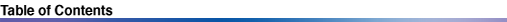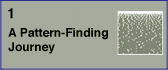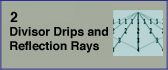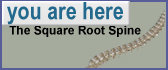3. The Square Root Spine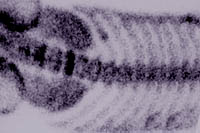A curved spine arches through the divisor plot: the square root boundary. It defines an axis of symmetry. The divisor plot is fundamentally two-dimensional, and this is because multiplication is a tango for two: all divisors come in pairs, and those pairs lie on either side of the spine. This fact is fundamental to much of the patterning in the divisor plot. The illustration below shows the beginning of the curve.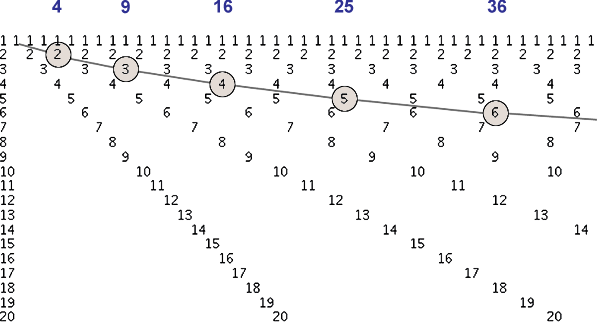In this image, the square roots of the first six perfect squares, (1, 4, 9, 16, 25, 36) are highlighted with circles. This boundary becomes increasingly horizontal at higher numbers. One way to determine whether an integer x is composite or not is to test whether it is evenly divisible by any integer i greater than 1 and less than the square root of x. It is not necessary to test for larger i since those are the companion divisors of the ones less than square root of x. Remember that all divisors come in conjugate pairs (i1 < square root of x, and i2 > square root of x ) except for the roots of perfect squares, in which case i = square root of x. This dual property of divisors is expressed in the symmetry of the square root boundary, which is the curve y = square root of x. The image below illustrates this idea: in order to check if a number is composite or prime, you only need to refer to the shaded area at the top.Notice that the number 23 has no divisors less than its square root (other than 1, which we don't count), and this is all that has to be checked to determine that it is prime. Wolfram describes a kind of quadratic sieve that can generate the prime numbers, using the integer points lying on the parabola x = y2. The image below shows the sieve. It was lifted from a paper by Abigail Kirk, who refers to it as a "visual sieve", because it lets the primes be picked out visually. Kirk references Yuri Matiyasevich and Boris Stechkin as the originators . To build the sieve, plot a parabola on a grid as shown below in image (a). On each arm of the parabola, plot all the points (i2, i) with i being a whole number, starting from 2, and stopping at some maximum value. Label the number using i. Then connect each pair of points occurring above and below the x-axis, as shown. The points where these lines intersect the x-axis correspond to composite numbers. Points where no lines cross are prime numbers.The first thing I noticed when I saw this graph was that if you overlay the bottom half of this graph onto the divisor plot, the bottom arm of the parabola happens to lie on the square root boundary, as shown in (b). Not surprisingly, there is a lot of crossing of lines going on at divisor locations. Perhaps there is more to be learned from studying this overlay. Symmetry The illustration below shows D36. Its divisor pairs are (1, 36) (2, 18) (3, 12) (4, 9). And it has a perfect square root: 6. Notice that the two numbers in each pair in this series converge towards the square root boundary.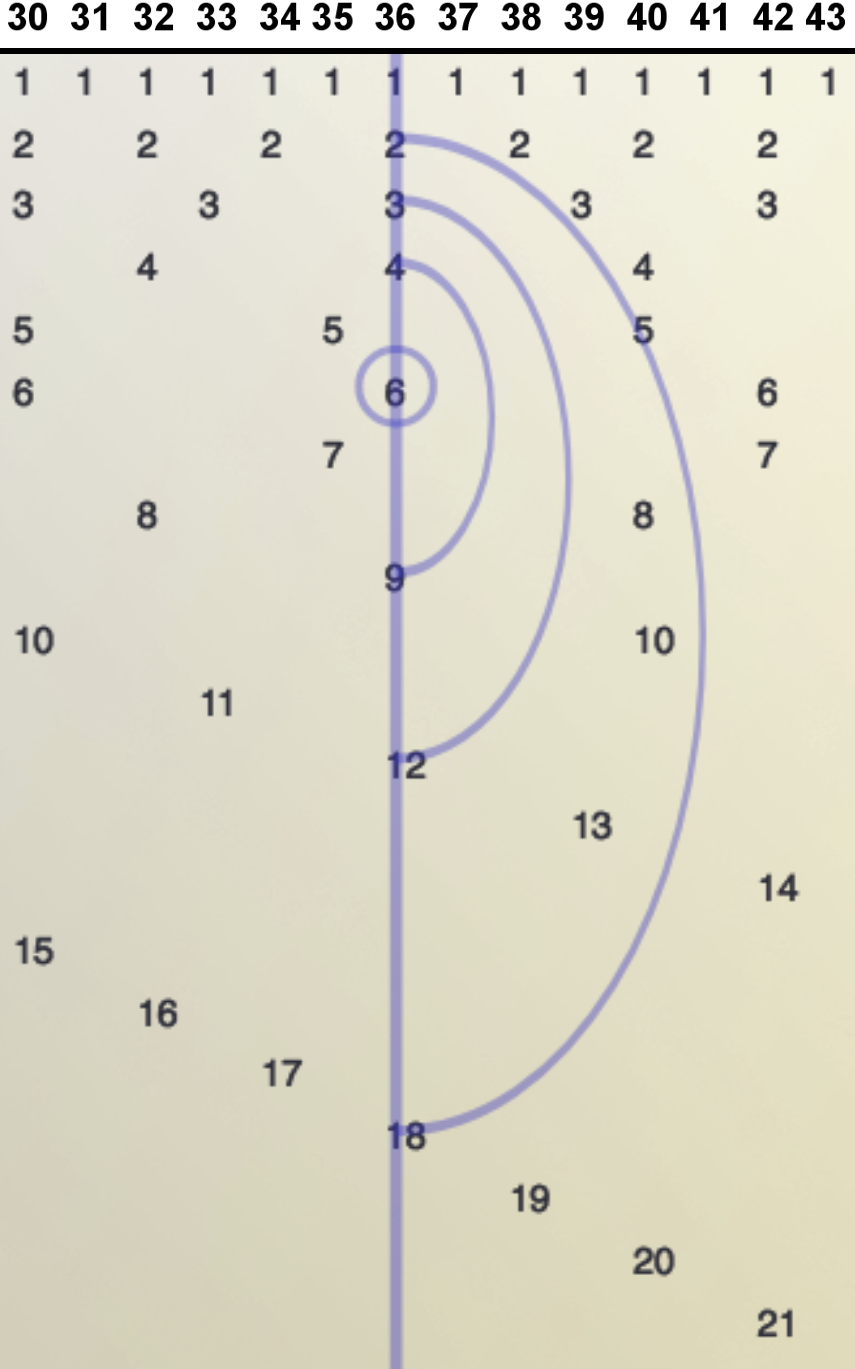This is just a hint of the kind of symmetry that becomes more complex at larger numbers. For instance, the image below shows the square root boundary at one million, with its square root 1000, indicated by the arrow.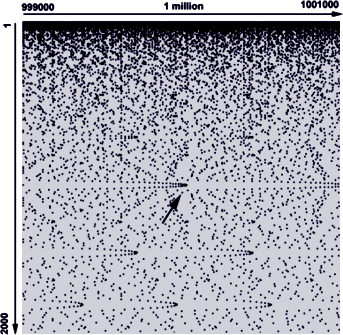You may notice a parabolic shape with its vertex located at the square root of a million. What's up with that? The next chapter is all about these peculiar shapes: the square root parabolas of the divisor plot